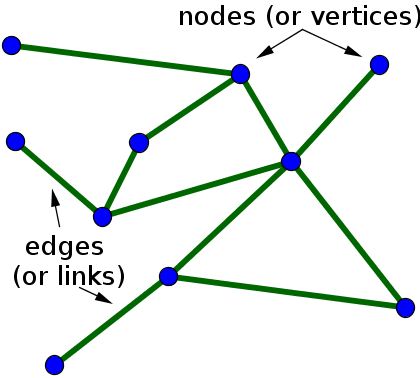# Math Insight

### Undirected graph definition

An undirected graph is graph, i.e., a set of objects (called vertices or nodes) that are connected together, where all the edges are bidirectional. An undirected graph is sometimes called an undirected network. In contrast, a graph where the edges point in a direction is called a directed graph.

When drawing an undirected graph, the edges are typically drawn as lines between pairs of nodes, as illustrated in the following figure.An undirected graph with 10 and 11 edges.

One can formally define an undirected graph as $G= (\mathcal{N},\mathcal{E})$, consisting of the set $\mathcal{N}$ of nodes and the set $\mathcal{E}$ of edges, which are unordered pairs of elements of $\mathcal{N}$.